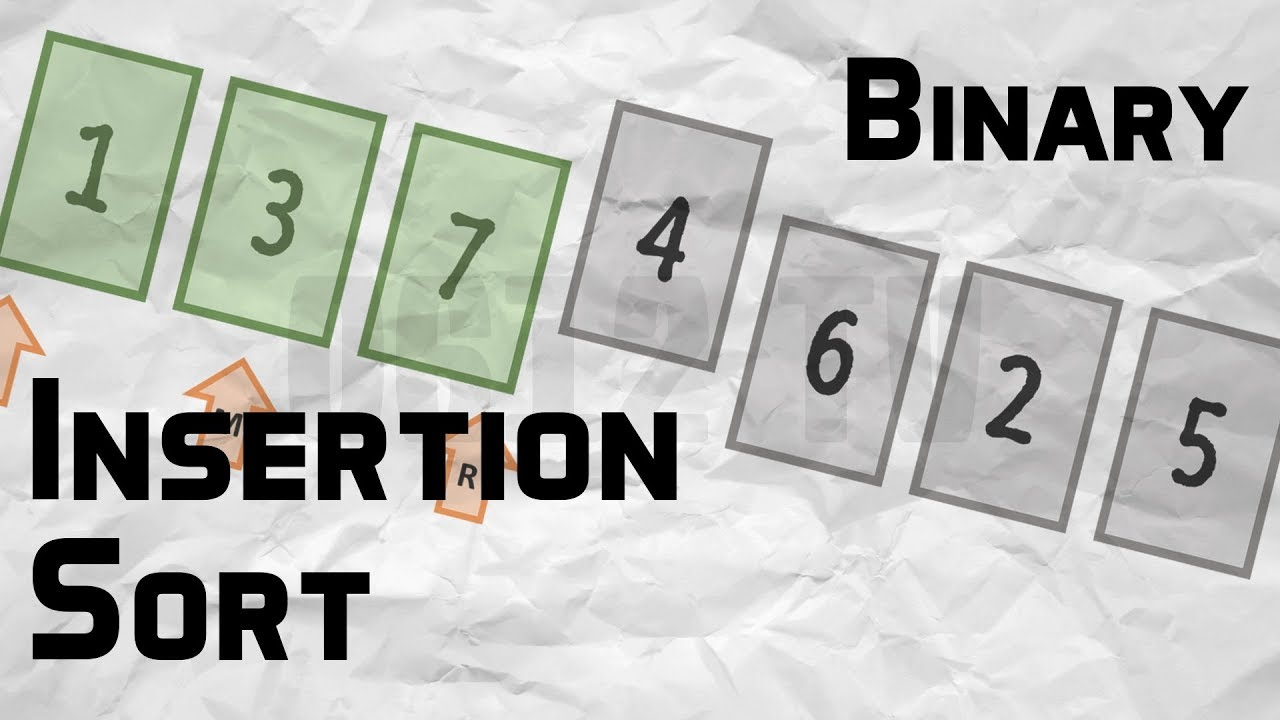# Which sorting algorithm makes minimum number of memory writes?

Which sorting algorithm makes minimum number of memory writes – Searching and Sorting – Minimizing the number of writes is useful when making writes to some huge data set is very expensive, such as with EEPROMs or Flash memory, where each write reduces the lifespan of the memory.

Minimizing the number of writes is useful when making writes to some huge data set is very expensive, such as with EEPROMs or Flash memory, where each write reduces the lifespan of the memory.

Among the sorting algorithms that we generally study in our data structure and algorithm courses,  Selection Sort makes least number of writes (it makes O(n) swaps).  But, Cycle Sort almost always makes less number of writes compared to Selection Sort.  In Cycle Sort, each value is either written zero times, if it’s already in its correct position, or written one time to its correct position. This matches the minimal number of overwrites required for a completed in-place sort.

##### You May Also Like## Binary Insertion Sort

Binary Insertion Sort – Searching and Sorting – We can use binary search to reduce the number of comparisons in normal insertion sort. Binary Insertion Sort find use binary search to find the proper location to insert the selected item at each iteration.

## Add 1 to a given number

Add 1 to a given number – Bit Algorithm – Add 1 to a given number write a program to add 1 to a given number. You are not allowed to use operators like ‘+’,